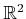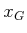#### Lyapunov functions in planning

Lyapunov functions are closely related to navigation functions and optimal cost-to-go functions in planning. In the optimal discrete planning problem of Sections 2.3 and 8.2, the cost-to-go values can be considered as a discrete Lyapunov function. By applying the computed actions, a kind of discrete vector field can be imagined over the search graph. Each applied optimal action yields a reduction in the optimal cost-to-go value, until 0 is reached at the goal. Both the optimal cost-to-go and Lyapunov functions ensure that the trajectories do not become trapped in a local minimum. Lyapunov functions are more general than cost-to-go functions because they do not require optimality. They are more like navigation functions, as considered in Chapter 8. The requirements for a discrete navigation function, as given in Section 8.2.2, are very similar to the positive definite condition given in this section. Imagine that the navigation function shown in Figure 8.3 is a discrete approximation to a Lyapunov function over. In general, a Lyapunov function indicates some form of distance to, although it may not be optimal. Nevertheless, it is based on making monotonic progress toward. Therefore, it may serve as a distance function in many sampling-based planning algorithms of Chapter 14. Since it respects the differential constraints imposed by the system, it may provide a better indication of how to make progress during planning in comparison to a Euclidean metric that ignores these considerations. Lyapunov functions should be particularly valuable in the RDT method of Section 14.4.3, which relies heavily on the distance function over.

Steven M LaValle 2020-08-14